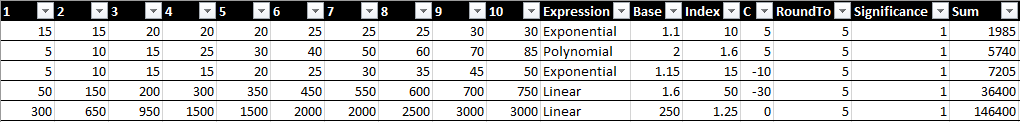# # Growth Rate Table

If there is one task that you will ABSOLUTELY spend your day thinking about, it is growth rate.

Growth Rate: How quickly a certain value grows in your system. A common example would be the function that controls how much XP the player needs every level.

Whether you need to figure out the amount of XP required to level up, or how much money the player should get for completing a quest, or the number of enemies to spawn in an encounter, it will most likely involve some increase in a number over time.

This is such a common task, that I have a short little cheat sheet that I use to bootstrap my systems. It is very simple, and it look like this:There are four components of this bootstrapping system:

1. Four types of basic Expressions
2. All built on the same four Terms
3. All in a table that allows for easy comparison
4. With some simple helper output and cleaning up.

## # Common Terms

There are four terms that are shared between all four expressions. Three of them are input by the user (C, Base, and Index), the other is not. The fourth term, N, is a derived value.

• C, the Constant that is added to the expression.
• Base, most often this acts as the Coefficient of the expression. I call it the Base as it is usually the number you want to "start" with, and then things go up from there.
• Index, this is usually the exponent of the function.
• N, this is the "level" or the "rank" of the growth formula.

## # The Expressions

I support four basic expressions, which in my experience covers basically every use case I ever have when it comes to growth formulas.

``````Linear = C + Base * Index * N

Polynomial = C + Base * N ^ Index

Exponential = C + Index * Base ^ N

Explosive = C + Base ^ (Index * N)
``````

## # A Live Example

Let's see this in a live example. Try playing around with the different values, and study the difference between the four types of expressions. They each grow at a different rate, and they each accomplish very different types of problems that you might need to solve.

Type 12345 678910
Linear 153045607590105120135150
Polynomial 10285280112147185226270316
Exponential 151501500150001.5e+51.5e+61.5e+71.5e+81.5e+91.5e+10
Explosive 10316100003.2e+51.0e+73.2e+81.0e+103.2e+111.0e+133.2e+14